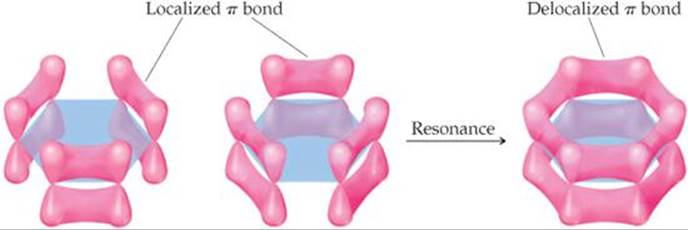# Why is the heat of hydrogenation of benzene less than expected?

Jun 29, 2017

The heat of hydrogenation is less than expected because benzene is stabilized by resonance.

#### Explanation:

Heats of hydrogenation

Cyclohexene has one double bond, and its heat of hydrogenation is -120 kJ/mol.

Benzene has three double bonds, so we might expect its heat of hydrogenation to be -360 kJ/mol.However, its measured heat of hydrogenation is only -208 kJ/mol.

Benzene is more stable than expected by 152 kJ/mol. This difference is called its resonance energy.

ResonanceWe can write two Lewis structures for benzene, differing only in the positions of the electrons.

Whenever we can do this, the correct structure is neither of the two. It is a resonance hybrid of them both.

Such a structure is more stable than either of the contributors, and the extra stabilization is called the resonance energy of the compound.

The orbital explanation

We can understand this in terms of the orbitals involved.

The carbon atoms in benzene are ${\text{sp}}^{2}$ hybridized, and the unhybridized ${\text{p}}_{z}$ orbitals overlap side-on to form the π-clouds of the $\text{C=C}$ double bonds.All six $\text{p}$ orbitals can overlap side-on to form a "delocalized" π cloud of electrons around the ring.

Whenever electrons can spread out or "delocalize", they are at lower energy levels.

The overlap may appear to be small in the above diagram.However, the computer-drawn picture above shows that $\text{2p}$ orbitals are really much "fatter" than the elongated tear-drop shapes we usually draw, and the carbon atoms are close enough to each other, so side-on overlap of the orbitals is quite significant.